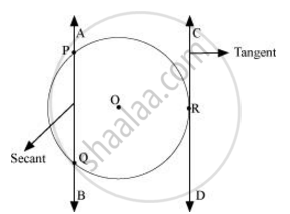Share

Books Shortlist

Draw a Circle and Two Lines Parallel to a Given Line Such that One is a Tangent and the Other, a Secant to the Circle. - CBSE Class 10 - Mathematics

Question

Draw a circle and two lines parallel to a given line such that one is a tangent and the other, a secant to the circle.

SolutionIt can be observed that AB and CD are two parallel lines. Line AB is intersecting the circle at exactly two points, P and Q. Therefore, line AB is the secant of this circle. Since line CD is intersecting the circle at exactly one point, R, line CD is the tangent to the circle.

Is there an error in this question or solution?

Video TutorialsVIEW ALL 

Solution Draw a Circle and Two Lines Parallel to a Given Line Such that One is a Tangent and the Other, a Secant to the Circle. Concept: Tangent to a Circle.
S